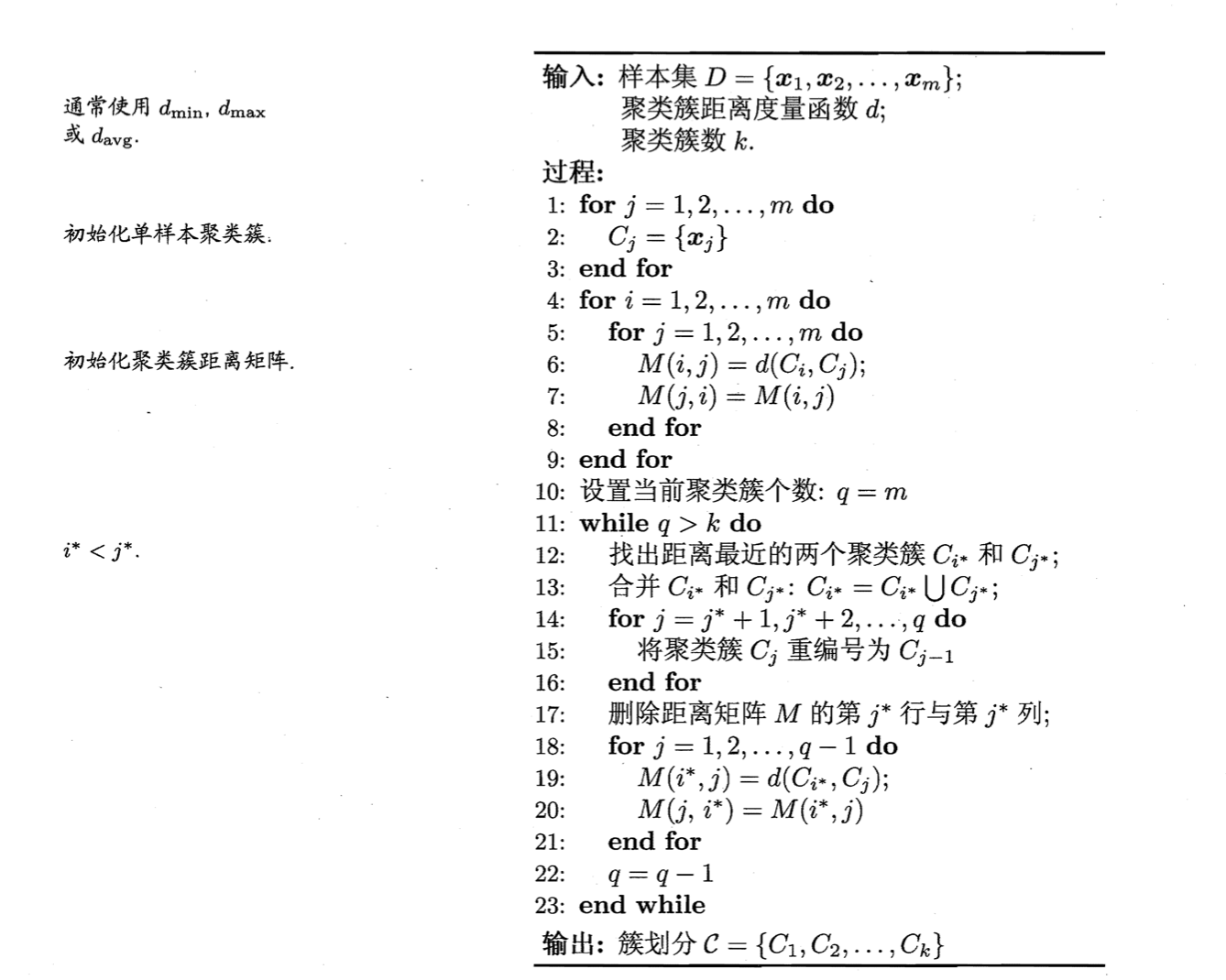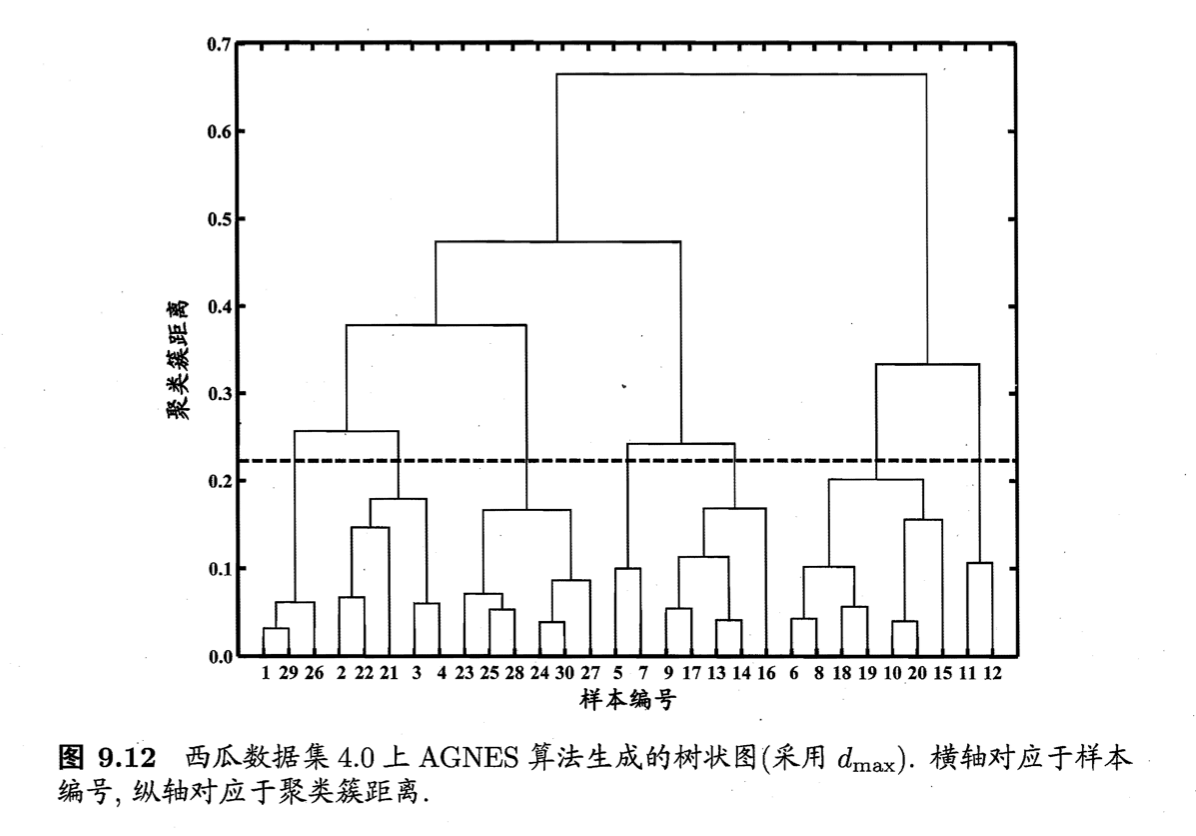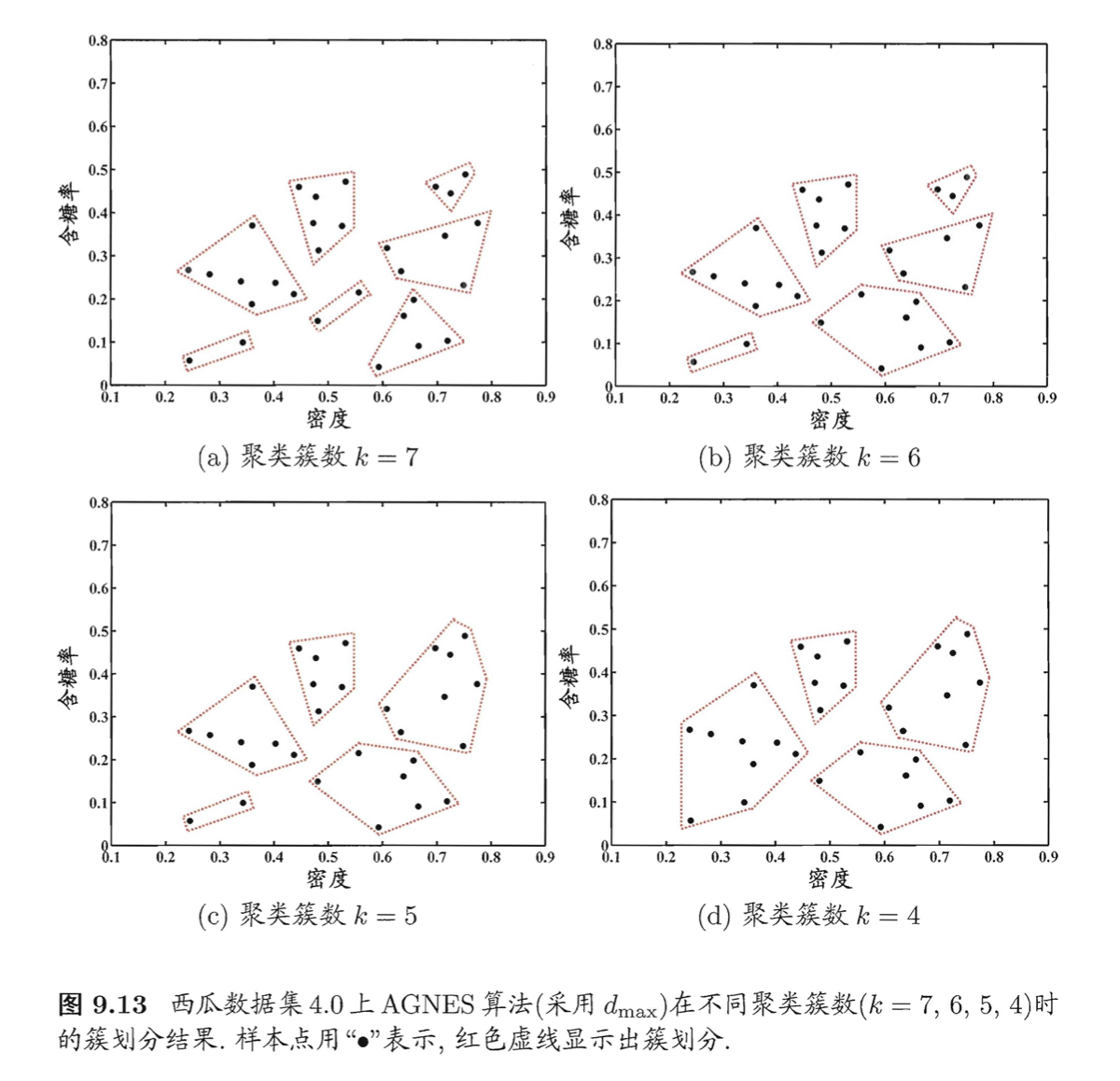# 【机器学习基础】第三十七课：聚类之层次聚类

## 层次聚类，AGNES算法

Posted by x-jeff on May 6, 2022

【机器学习基础】系列博客为参考周志华老师的《机器学习》一书，自己所做的读书笔记。

# 1.层次聚类

AGNES（AGglomerative NESting）是一种采用自底向上聚合策略的层次聚类算法。它先将数据集中的每个样本看作一个初始聚类簇，然后在算法运行的每一步中找出距离最近的两个聚类簇进行合并，该过程不断重复，直至达到预设的聚类簇个数。例如，给定聚类簇$C_i$与$C_j$，可通过下面的式子来计算距离：

$最小距离：d_{min}(C_i,C_j)=\min \limits_{\mathbf{x} \in C_i, \mathbf{z}\in C_j} \text{dist}(\mathbf{x},\mathbf{z}) \tag{1}$ $最大距离：d_{max}(C_i,C_j)=\max \limits_{\mathbf{x} \in C_i, \mathbf{z}\in C_j} \text{dist}(\mathbf{x},\mathbf{z}) \tag{2}$ $平均距离：d_{avg}(C_i,C_j)=\frac{1}{\lvert C_i \rvert \lvert C_j \rvert} \sum_{\mathbf{x} \in C_i} \sum_{\mathbf{z}\in C_j} \text{dist}(\mathbf{x},\mathbf{z}) \tag{3}$

AGNES算法描述如下图所示：